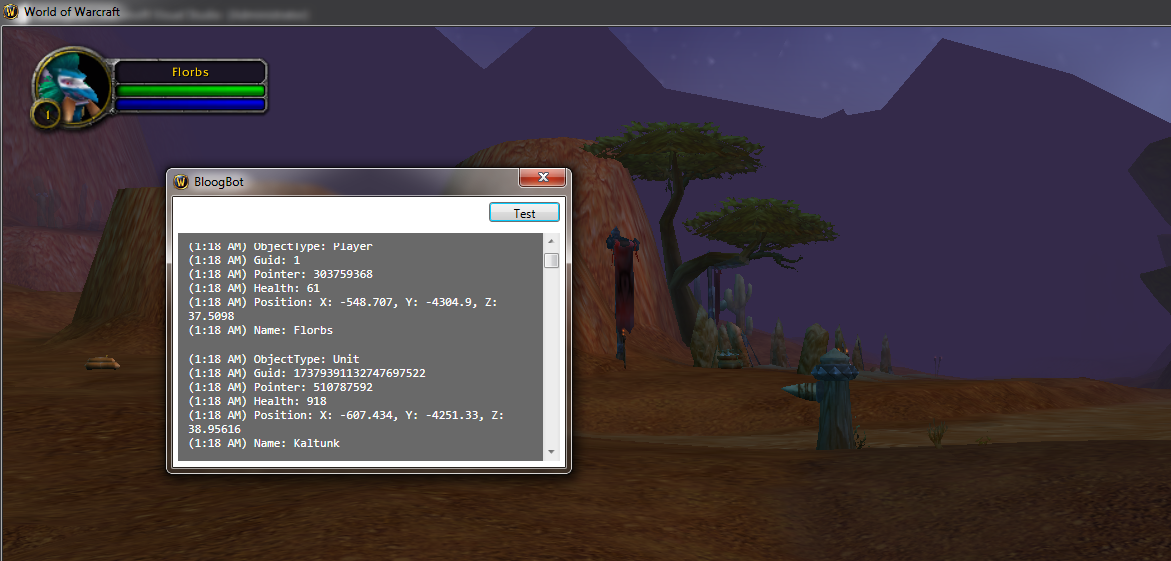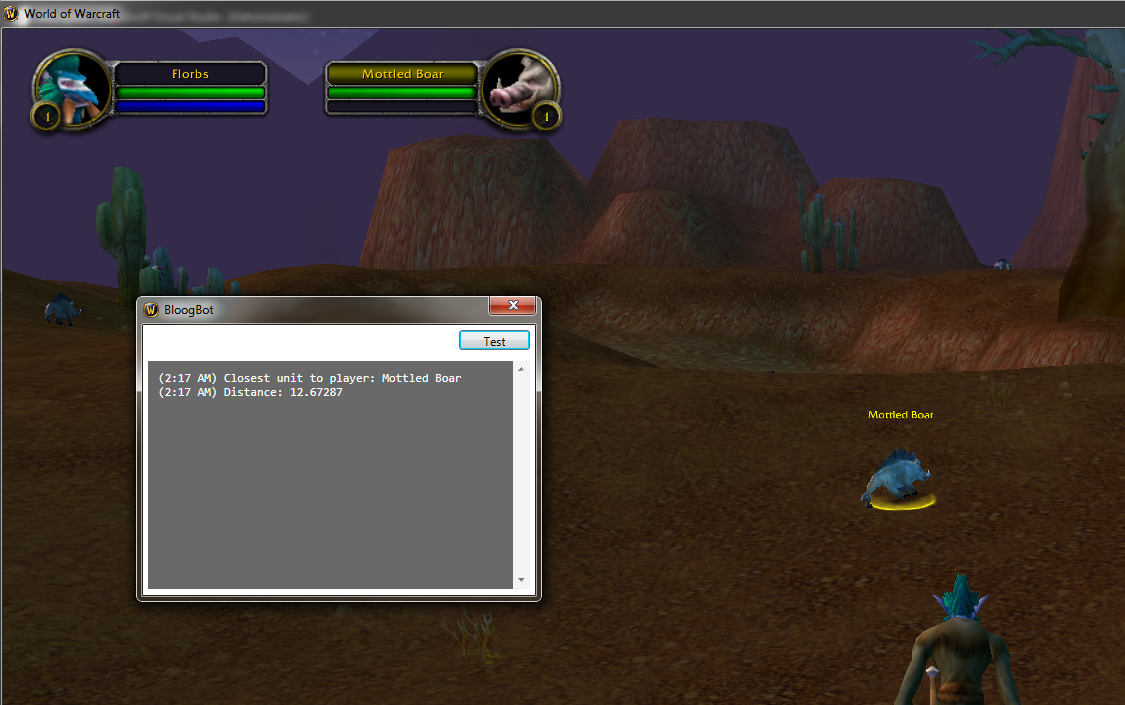# Bloog Bot

Chapter 9
Drew Kestell, 2022

## Position

The next thing we're going to do is implement the Player object type. There is actually an important distinction that we need to make - there can be many Players in the world, but only one LocalPlayer (you!). SO we're going to make two new classes - WoWPlayer which inherits from WoWUnit, and LocalPlayer which inherits from WoWPlayer. Both classes will stay very simple for now:

```class WoWPlayer : WoWUnit
{
internal WoWPlayer(IntPtr pointer, ulong guid, ObjectType objectType)
: base(pointer, guid, objectType)
{
}
}
```
```class LocalPlayer : WoWPlayer
{
internal LocalPlayer(IntPtr pointer, ulong guid, ObjectType objectType)
: base(pointer, guid, objectType)
{
}
}
```

We're also going to have to modify the `Callback` of `EnumerateVisibleObjects` in the `ObjectManager` class to handle these new object types. Recall that `EnumerateVisibleObjects` will call `Callback` internally, passing in the filter parameter, and the guid of the object. Also recall that we've already added a function to get the guid of the currently logged in player. So all we need to do is compare the guid passed to our Callback function with the result of `GetPlayerGuid` to determine when we've found the local player. If the guid is different, we've found some other player! First add a property on the `ObjectManager` class to store a reference to the local player:

```public static LocalPlayer Player { get; private set; }
```

Then modify the `Callback` method to look compare the guids accordingly, and set the Player field:

```static int Callback(int filter, ulong guid)
{
var pointer = Functions.GetObjectPtr(guid);
var objectType = (ObjectType)MemoryManager.ReadByte(
OBJECT_TYPE_OFFSET)
);

switch (objectType)
{
case ObjectType.Player:
if (guid == Functions.GetPlayerGuid())
{
var player = new LocalPlayer(pointer, guid, objectType);
Player = player;
}
else
ObjectsBuffer.Add(new WoWPlayer(pointer, guid, objectType));
break;
case ObjectType.Unit:
Objects.Add(new WoWUnit(pointer, guid, objectType));
break;
default:
Objects.Add(new WoWObject(pointer, guid, objectType));
break;
}

return 1;
}
```

There's one quirk that we need to fix related to the `Name` property on the `WoWUnit` class. We access a Player's name in a different way. So we're going to modify the `Name` property on the `WoWUnit` class to make it virtual, then we'll override that method in the `WoWPlayer` class. I haven't looked too closely at this section of the decompiled code from the WoW client, but I assume all player names are stored in a specific area of memory, and non-player units' names are stored elsewhere. We have the base address of that player name collection. We also know the offset between names. So we start at the base address, then walk the collection until we find the guid of the Player, then we read a string from memory at that location. Here's the code for the `WoWPlayer` class:

```class WoWPlayer : WoWUnit
{
// NAME
const int NAME_BASE_OFFSET = 0xC0E230;
const int NEXT_NAME_OFFSET = 0xC;
const int PLAYER_NAME_OFFSET = 0x14;

internal WoWPlayer(IntPtr pointer, ulong guid, ObjectType objectType)
: base(pointer, guid, objectType)
{
}

internal override string Name
{
get
{
var namePtr = MemoryManager.ReadIntPtr((IntPtr)NAME_BASE_OFFSET);
while (true)
{

if (nextGuid != Guid)
else
break;
}
}
}
}
```

Next we're going to start talking about positions in 3D space. It's very common in 3D games to use a 3D coordinate system to store the relative position of objects in the world. A basic understanding of geometry is necessary before jumping into the operations we'll be making in 3D coordinate space, so if this is new to you, take a look at this article.

Our bot needs to be able to move around, so eventually we're going to need to know the player's position in 3D space, as well as the position of all units around the player. Let's do that now. Unlike the Name property, both `WoWUnit` and `WoWPlayer` find their positions in the same relative place in memory, so we should add the new code to the base `WoWUnit` class. We'll most often be interested in all three dimensions at the same time (x, y, and z), so instead of implementing separate properties for each, let's create a `Position` class to encapsulate all three dimensions. Let's also have the `Position` class override the `ToString()` method so we can easily print a unit's position for debugging purposes. Here's the code:

```class Position
{
internal Position(float x, float y, float z)
{
X = x;
Y = y;
Z = z;
}

internal float X { get; }

internal float Y { get; }

internal float Z { get; }

public override string ToString() => \$"X: {X}, Y: {Y}, Z: {Z}";
}
```

This is our first time reading a `float` from memory, so we'll need a new method on the `MemoryManager` class:

```[HandleProcessCorruptedStateExceptions]
{
try
{
}
catch (AccessViolationException ex)
{
Console.WriteLine("Access Violation on " + address + " with type Float");
return default;
}
}
```

Finding the x, y, and z coordinates of a unit is actually quite simple. Each coordinate is simply stored at an offset relative to the base address of the unit:

```class WoWUnit : WoWObject
{
...

const int POS_X_OFFSET = 0x9B8;
const int POS_Y_OFFSET = 0x9BC;
const int POS_Z_OFFSET = 0x9C0;

internal WoWUnit(IntPtr pointer, ulong guid, ObjectType objectType)
: base(pointer, guid, objectType)
{
}

...

internal Position Position
{
get
{

return new Position(x, y, z);
}
}
}
```

Like usual, let's test this by modifying our `Test` method in the UI to print the position of all units. You'll see something like this:Now that we know the player's position, and the positions of all units around the player, we can perform all sorts of useful calculations. A simple example would be finding the unit closest to the player. First, let's make a quick fix to the `ObjectManager` class. Consider the following code:

```internal static IEnumerable<WoWUnit> Units => Objects.OfType<WoWUnit>();
```

The `Units` property is actually going to return units AND players, because a `WoWPlayer` is actually a specific type of `WoWUnit`. It would be more useful if we could get ONLY units, excluding the players. Let's change this property to be more specific (notice we need to to call `ToList()` here - the way LINQ does deferred execution here will screw up the cast from `WoWObject` to `WoWUnit` without it):

```internal static IEnumerable<WoWUnit> Units => Objects.OfType<WoWUnit>().Where(o => o.ObjectType == ObjectType.Unit).ToList();
```

So, in order to find the closest NPC to the player, we first need the ability to determine the distance between two positions. Let's make that it's own method on the `Position` class. Most people are probably familiar with using the pythagorean theorem to find the length of the hypotenuse of a triangle in 2D space. The technique is pretty much the same for 3D space. First we're going to find the deltas of all three coordinates, then we'll apply the pythagorean theorem.

```internal float DistanceTo(Position position)
{
var deltaX = X - position.X;
var deltaY = Y - position.Y;
var deltaZ = Z - position.Z;

return (float)Math.Sqrt(deltaX * deltaX + deltaY * deltaY + deltaZ * deltaZ);
}
```

Now that we can find the distance between two positions, in order to determine the closest unit to the player, we can simply order the units by distance to the player in ascending order, then take the first element. Let's test it:

```void Test()
{
ObjectManager.EnumerateVisibleObjects();

var player = ObjectManager.Player;

var units = ObjectManager.Units;
var closestUnit = units.OrderBy(u => u.Position.DistanceTo(player.Position)).First();

Log(\$"Closest unit to player: {closestUnit.Name}");
Log(\$"Distance: {closestUnit.Position.DistanceTo(player.Position)}");
}
```

Fire up your client and give it a go. You should see something like:Considering a bot spends most of its time running between enemies and laying waste to them, being able to find the nearest enemy is a fundamental piece of behavior. In the next chapter we'll see how to make the bot move.Subscribe to the RSS feed to keep up with new chapters as they're released